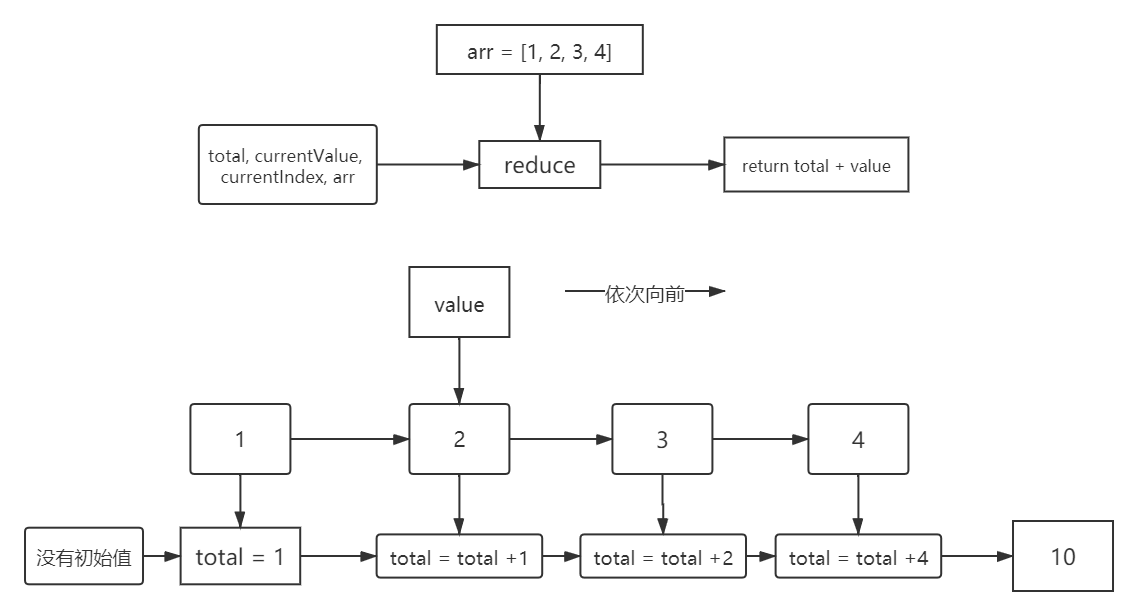## # 1. 高阶函数

• 接受一个或多个函数作为输入
• 输出一个函数

### # 1.1 map

`map`方法接收一个函数作为参数 ，遍历数组，并且返回一个新的数组，新的数组里的每个元素都执行`map`传入的函数。

``````let arr = [1, 2, 3, 4];
let arr1 = arr.map(item => item * 2)
console.log(arr1);// [2, 4, 6, 8]
``````
1
2
3

`注意`：如果传入的参数没有返回值，则数组的每一项都会是`undefind`

#### #经典题目

``````console.log(['1','2','3'].map(parseInt));
``````
1

`parseInt()` 函数可解析一个字符串，并返回一个整数。

• 如果 字符串 以 "0x" 开头，`parseInt()` 会把 其余部分解析为十六进制的整数。
• 如果字符串以 0 开头，把其余部分解析为八进制或十六进制的数字。
• 如果字符串以 1 ~ 9 的数字开头，`parseInt()`将把它解析为十进制的整数

`注意`：基数可不是默认十进制噢！

``````parseInt('1', 0)
parseInt('2', 1)
parseInt('3', 2)
``````
1
2
3

`注意`：如果字符串的第一个字符不能被转换为数字，那么`parseInt()`会返回 NaN。

`parseInt()`还有很多值得注意的问题，可以使用搜索引擎再了解以下

### # 1.2 filter

`filter`方法接收一个函数作为参数，通过这个函数来指定筛选数组的规则，最后返回满足规则的新数组

currentValue 必须。当前元素的值
index 可选。当前元素的索引值
arr 可选。当前元素属于的数组对象

`注意`

• 不会检测空数组
• 不会改变原始数组
``````let arr = [1, 2, 3, 4, 5, 6, 7, 8, 9]
let arr1 = arr.filter(num => {
return num > 5
})
console.log(arr1);// [6, 7, 8, 9]
``````
1
2
3
4
5

### # 1.3 reduce

`reduce`能做的事情很多，但是我们平时都使用for循环之类的方法代替了，但是`reduce`真的高逼格

``````array.reduce(function(total, currentValue, currentIndex, arr), initialValue)
``````
1

`w3school`中给出的reduce语法，这里我们常用的只有前面两个

total 必需。初始值, 或者计算结束后的返回值。
currentValue 必需。当前元素
``````let arr = [1, 2, 3, 4]
let sum = arr.reduce((value, item) => {
console.log(value, item);
// 1 2   3 3  6 4
return value + item
})
console.log(sum);// 10
``````
1
2
3
4
5
6
7``````let arr = [1,1,2,3,4,2,5,4];
let unique = arr.reduce(function (prev, item) {
prev.indexOf(item) === -1 && prev.push(item);
return prev;
}, []);
console.log(unique); // [1, 2, 3, 4, 5]
``````
1
2
3
4
5
6

`ruduce`的用法远不止这些，有兴趣的可以再了解以下~

## # 2. AOP 面向切面编程

• 下面我们要实现在函数==执行前==输出提示信息
``````function say(who) {
console.log(who + '：函数执行了');
}
Function.prototype.before = function(callback) {
return (...args) => {
callback()
this(...args)
}
}
let whoSay = say.before(function() {
console.log('你要被调用了');
})
whoSay('ljc')
// 你要被调用了
// ljc：函数执行了
``````
1
2
3
4
5
6
7
8
9
10
11
12
13
14
15

## # 3. 偏函数

• 实现3个数求和
``````function sum(a, b, c){
return a + b + c;
}
sum(1, 2, 3) // 6
``````
1
2
3
4

``````function sum(a, b, c) {
return a + b + c
}

function partial(sum, c) {
return function (a, b) {
return sum(a, b, c)
}
}
let partialSum = partial(sum, 3)// -> 6
``````
1
2
3
4
5
6
7
8
9
10

`Function.prototype.bind` 函数就是一个偏函数的典型代表，它接受的第二个参数开始，为预先添加到绑定函数的参数列表中的参数

## # 4. 函数柯里化

``````function add(a, b) {
return a + b;
}
add(1, 2) // 3  普通做法 一次传入两个参数

// 假设有一个 curring 函数可以做到柯里化
function curring(){}
curring(1)(2) // 我们通过这样的方式来接受参数，这样就实现了柯里化
``````
1
2
3
4
5
6
7
8

``````function curring(x) {
return return y => x + y
}
curring(1)(2)  // => 3
``````
1
2
3
4

### # 4.1 函数柯里化的作用

#### # 4.1.1 参数复用

``````function myInfo(inf, name, age) {
return `\${inf}：\${name}\${age}`
}
const myInfo1 = myInfo('个人信息', 'ljc', '19')
console.log(myInfo1); // 个人信息：ljc19
``````
1
2
3
4
5

``````function myInfoCurry(inf) {
return (name, age) => {
return `\${inf}：\${name}\${age}`
}
}
let myInfoName = myInfoCurry('个人信息')
const myInfo1 = myInfoName('ljc', '19')
const myInfo2 = myInfoName('ljcc','19')
console.log(myInfo2); // 个人信息：ljcc119
console.log(myInfo1); // 个人信息：ljc19
``````
1
2
3
4
5
6
7
8
9
10

``````let myInfoSex = myInfoCurry('爱好')
const myInfo3 = myInfoSex('看球赛','听歌')
console.log(myInfo3); // 爱好：看球赛听歌
``````
1
2
3

#### # 4.1.2 提前返回

``````const whichEvent = (function () {
return function (ele, type, listener, useCapture) {
listener.call(ele, e)
}, useCapture)
}
} else if (window.attachEvent) {
return function (ele, type, handler) {
ele.attachEvent('on' + type, function (e) {
handler.call(ele, e)
})
}
}
})()
``````
1
2
3
4
5
6
7
8
9
10
11
12
13
14
15

#### # 4.1.3 延迟执行

``````function add(...args) {
let inner = function () {
args.push(...arguments);
inner.toString = function () {
return args.reduce((prev, cur) => {
return prev + cur
})
}
return inner
}
return inner
}
``````
1
2
3
4
5
6
7
8
9
10
11
12
13

lastUpdate: 5/22/2022, 4:34:51 PM